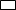Chapter 4.3, Problem 19EElementary Geometry For College St...

7th Edition
Alexander + 2 others
ISBN: 9781337614085

Solutions

Chapter
SectionElementary Geometry For College St...

7th Edition
Alexander + 2 others
ISBN: 9781337614085
Textbook Problem

Given:ABCD (not shown) with AB = 8 and BC = 6; M and N are the midpoints of sides A B ¯  and  B C ¯ , respectively. Find: MN

To determine

To find:

MN of the given rectangle.

Explanation

The basic properties of rectangle:

1) All the properties of a parallelogram apply (the ones that matter here are parallel sides, opposite sides are congruent, and diagonals bisect each other).

2) All angles are right angles by definition.

3) The diagonals are congruent.

Calculation:

Given: In Rectangle ABCD, AB = 8 and BC = 6.

M and N are the midpoints of sides AB¯ and BC¯, respectively.

Consider the rectangle.

To find MN:

From the property, in rectangle the diagonals bisect each other.

In rectangle ABCD

AM=MB=AB2=82=4

NC

Still sussing out bartleby?

Check out a sample textbook solution.

See a sample solution

The Solution to Your Study Problems

Bartleby provides explanations to thousands of textbook problems written by our experts, many with advanced degrees!

Get Started

In Exercises 7-28, perform the indicated operations and simplify each expression. 19. 4x222x2+7+2x2+7

Applied Calculus for the Managerial, Life, and Social Sciences: A Brief Approach

Evaluate the integral. 45. x5ex3dx

Calculus: Early Transcendentals

In Problems 1-12, find the derivative of each function. 12.

Mathematical Applications for the Management, Life, and Social Sciences

Simplify: 918

Elementary Technical Mathematics

limx0cscx= a) 0 b) c) d) does not exist

Study Guide for Stewart's Single Variable Calculus: Early Transcendentals, 8th